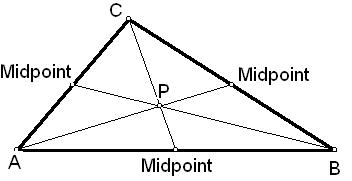# Centroid of a triangle Calculator

Calculator finds the coordinates on the centroid of a triangle for entered coordinates of the 3-vertices.
 X1: Y1: X2: Y2: X3: Y3:

 Centroid(P)
The point through which all the three medians of a triangle pass is called Centroid of triangle. The each median connecting a vertex with the midpoint of the opposite side. It is also called the center-of-gravity of the triangle or as the barycent. The coordinates of the centroid are the average of the vertices-coordinates.
Centroid Triangle Formula:
(( x1 + x2 + x3 )/3 , (y1 + y2 + y3 )/3).